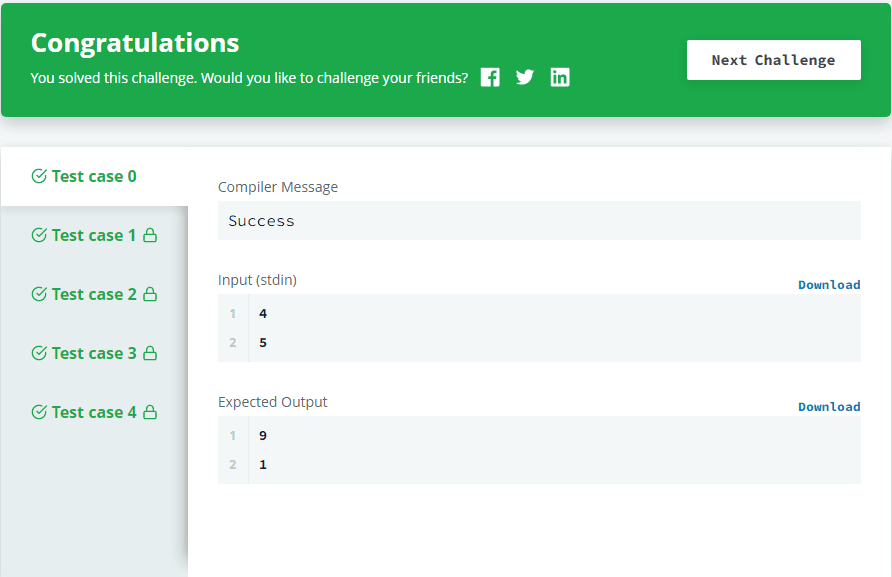# Pointer in C++ - Hackerrank Solution

## Pointer in C++ - Hackerrank Solution

Hope you are having a good day coders,

Today we will learn about Pointers and using that we will be solving Pointer in C++ Hackerrank Problem.

Pointers are one of the crucial components in C++, so it is must to know about this topic.

So, Without wasting your time let's jump into the solution.

## Problem

A Pointer in C++ is used to share a memory address among different contexts (primarily functions). They are used whenever a function needs to modify the content of a variable, but it does not have ownership.

In order to access the memory address of a variable, val, prepend it with & sign. For example, &val returns the memory address of val.

This memory address is assigned to a pointer and can be shared among functions. For example, int*p = &val assigns the memory address of val to pointer p. To access the content of the memory pointed to, prepend the variable name with a *. For example, *p will return the value stored in val and any modification to it will be performed on val.

```void increment (int *v) {
(*v)++;
}

int main() {
int a;
scanf("%d", &a);
increment(&a);
printf("%d", a);
return 0;
}
```

## Function Description

Complete the update function in the editor below.

update has the following parameters:

• int*a: an integer
• int*b: an integer

## Returns

The function is declared with a void return type,so there is no value to return. Modify the values in memory so that a contains their sum and b contains their absoluted difference.

• a' = a + b
• b' = |a - b|

## Input Format

Input will contain two integers, a and b, separated by a newline.

```4
5
```

```9
1
```

## Explanation

a' = 4 + 5 = 9

b' = | 4 - 5 | = 1

## Solution - Pointer in C++

```#include <stdio.h>

void update(int *a,int *b) {
// Complete this function
int temp = *a;
*a = *a + *b;
*b = temp - *b;

if (*b < 0)
{
*b = -(*b);
}
}

int main() {
int a, b;
int *pa = &a, *pb = &b;

scanf("%d %d", &a, &b);
update(pa, pb);
printf("%d\n%d", a, b);

return 0;
}
```Pointer in C++ - Hackerrank SolutionPointer in C++ - Result

## Conclusion

So, coders today we have learned about what are Pointers in C++ and how to use them in our program. If you have any doubt regarding the problem (Pointer in C++), feel free to contact in the Comment section.